# Teutonic Order and Livonian Order

#### unmerged(54293)

##### Second Lieutenant
The Order's monarchlist, still in vanilla form, is messed up a bit - one grandmaster is missing and there are several spelling mistakes. Worst, however, is that it does not contain the historical grandmasters after Albrecht of Brandenburg and his secularization of the Teutonic Order's lands.

That's why I compiled an updated list. There are two remaining issues which should be discussed: First, while German sources give the names of certain grandmasters with the land of their origion (e.g. "of Brandenburg-Ansbach", "of Österreich"), other languages tend to use dynasties instead (e.g. "of Hohenzollern", "of Habsburg"). I think we could go either way, but it should be uniform. Second, the problem of Albrecht of Brandenburg-Ansbach/Hohenzollern. If a player decides not to secularize and stay as the order, Albrecht would not be deposed as grandmaster in 1525 and could - most likely - rule until his death in 1568. Therefore, two historical grandmasters between 1525 and 1568 have to be skipped from the list. I think this can not be avoided, as there was no reason for Albrecht to abdicate in 1525 if he chose not to convert to Protestantism.

So, finally, here is the list.

Code:
``````# Teutonic Order #

# Grandmasters of the Teutonic Order. Original by Paradox, enhanced by Todor. #

historicalmonarch = {
id = { type = 6 id = 075500 }
name = "Michael Küchmeister von Sternberg"
startdate = {
year = 1414
}
deathdate = {
day = 10
month = march
year = 1422
}
DIP = 5
MIL = 5
dormant = no
}
historicalmonarch = {
id = { type = 6 id = 075501 }
name = "Paul von Rusdorf"
startdate = {
day = 10
month = march
year = 1422
}
deathdate = {
day = 2
month = january
year = 1441
}
DIP = 5
MIL = 6
dormant = no
}
historicalmonarch = {
id = { type = 6 id = 075502 }
startdate = {
day = 2
month = january
year = 1441
}
deathdate = {
day = 7
month = november
year = 1449
}
DIP = 5
MIL = 6
dormant = no
}
historicalmonarch = {
id = { type = 6 id = 075503 }
name = "Ludwig von Erlichshausen"
startdate = {
day = 7
month = november
year = 1449
}
deathdate = {
day = 4
month = april
year = 1467
}
DIP = 2
MIL = 2
dormant = no
}
historicalmonarch = {
id = { type = 6 id = 075504 }
name = "Heinrich Reuß von Plauen"
startdate = {
day = 4
month = april
year = 1467
}
deathdate = {
day = 2
month = january
year = 1470
}
DIP = 6
MIL = 5
dormant = no
}
historicalmonarch = {
id = { type = 6 id = 075505 }
name = "Heinrich Reffle von Richtenberg"
startdate = {
day = 2
month = january
year = 1470
}
deathdate = {
day = 4
month = august
year = 1477
}
DIP = 5
MIL = 5
dormant = no
}
historicalmonarch = {
id = { type = 6 id = 075506 }
name = "Martin Truchseß von Wetzhausen"
startdate = {
day = 4
month = august
year = 1477
}
deathdate = {
day = 3
month = january
year = 1489
}
DIP = 5
MIL = 6
dormant = no
}
historicalmonarch = {
id = { type = 6 id = 075507 }
name = "Johann von Tiefen"
startdate = {
day = 3
month = january
year = 1489
}
deathdate = {
day = 25
month = august
year = 1497
}
DIP = 4
MIL = 3
dormant = no
}
historicalmonarch = {
id = { type = 6 id = 075508 }
name = "Friedrich von Wettin"
startdate = {
day = 25
month = august
year = 1497
}
deathdate = {
day = 14
month = december
year = 1510
}
DIP = 5
MIL = 5
dormant = no
}
historicalmonarch = {
id = { type = 6 id = 075509 }
name = "Albrecht von Hohenzollern"
startdate = {
day = 14
month = december
year = 1510
}
deathdate = {
day = 20
month = march
year = 1568
}
DIP = 7
MIL = 5
dormant = no
remark = "Had Albrecht not decided to convert to Protestantism and secularize the Order's lands, he could have ruled
as Grandmaster until his death in 1568."
}
historicalmonarch = {
id = { type = 6 id = 075510 }
name = "Georg Hundt von Weckheim"
startdate = {
day = 20
month = march
year = 1568
}
deathdate = {
day = 17
month = march
year = 1572
}
DIP = 4
MIL = 4
dormant = no
}
historicalmonarch = {
id = { type = 6 id = 075511 }
name = "Heinrich von Bobenhausen"
startdate = {
day = 17
month = march
year = 1572
}
deathdate = {
year = 1590
}
DIP = 4
MIL = 5
dormant = no
}
historicalmonarch = {
id = { type = 6 id = 075512 }
name = "Maximilian von Habsburg"
startdate = {
year = 1590
}
deathdate = {
day = 2
month = november
year = 1618
}
DIP = 5
MIL = 3
dormant = no
}
historicalmonarch = {
id = { type = 6 id = 075513 }
name = "Karl von Habsburg"
startdate = {
day = 2
month = november
year = 1618
}
deathdate = {
day = 28
month = december
year = 1624
}
DIP = 4
MIL = 4
dormant = no
}
historicalmonarch = {
id = { type = 6 id = 075514 }
name = "Johann Eustach von Westernach"
startdate = {
day = 28
month = december
year = 1624
}
deathdate = {
day = 25
month = october
year = 1627
}
DIP = 4
MIL = 4
dormant = no
}
historicalmonarch = {
id = { type = 6 id = 075515 }
name = "Johann Kaspar von Stadion"
startdate = {
day = 25
month = october
year = 1627
}
deathdate = {
day = 21
month = november
year = 1641
}
DIP = 5
MIL = 5
dormant = no
}
historicalmonarch = {
id = { type = 6 id = 075516 }
name = "Leopold Wilhelm von Habsburg"
startdate = {
day = 21
month = november
year = 1641
}
deathdate = {
day = 20
month = november
year = 1662
}
DIP = 7
MIL = 7
dormant = no
}
historicalmonarch = {
id = { type = 6 id = 075517 }
name = "Karl Joseph von Habsburg"
startdate = {
day = 20
month = november
year = 1662
}
deathdate = {
day = 27
month = january
year = 1664
}
DIP = 2
MIL = 2
dormant = no
remark = "Died as a youth, could not fulfill his duties."
}
historicalmonarch = {
id = { type = 6 id = 075518 }
name = "Johann Caspar von Ampringen"
startdate = {
day = 27
month = january
year = 1664
}
deathdate = {
day = 9
month = september
year = 1684
}
DIP = 4
MIL = 3
dormant = no
}
historicalmonarch = {
id = { type = 6 id = 075519 }
name = "Ludwig Anton von Pfalz-Neuburg"
startdate = {
day = 9
month = september
year = 1684
}
deathdate = {
day = 4
month = may
year = 1694
}
DIP = 5
MIL = 4
dormant = no
}
historicalmonarch = {
id = { type = 6 id = 075520 }
name = "Franz Ludwig von Pfalz-Neuburg"
startdate = {
day = 4
month = may
year = 1694
}
deathdate = {
day = 6
month = april
year = 1732
}
DIP = 5
MIL = 5
dormant = no
}
historicalmonarch = {
id = { type = 6 id = 075521 }
name = "Klemens August von Wittelsbach"
startdate = {
day = 6
month = april
year = 1732
}
deathdate = {
day = 6
month = february
year = 1761
}
DIP = 4
MIL = 4
dormant = no
remark = "Also Elector-Archbishop of Köln, id = 070015."
}
historicalmonarch = {
id = { type = 6 id = 075522 }
name = "Karl Alexander von Lothringen"
startdate = {
day = 6
month = february
year = 1761
}
deathdate = {
day = 4
month = july
year = 1780
}
DIP = 4
ADM = 7 # Successful governor of the Low Countries.
MIL = 4
dormant = no
}
historicalmonarch = {
id = { type = 6 id = 075523 }
name = "Maximilian Franz von Habsburg"
startdate = {
day = 4
month = july
year = 1780
}
deathdate = {
day = 26
month = july
year = 1801
}
DIP = 5
MIL = 2
dormant = no
}
historicalmonarch = {
id = { type = 6 id = 075524 }
name = "Karl Ludwig von Habsburg"
startdate = {
day = 26
month = july
year = 1801
}
deathdate = {
year = 1804 # Resigned.
}
DIP = 4
MIL = 7 # Won against Napoleon at Aspern.
dormant = no
}
historicalmonarch = {
id = { type = 6 id = 075525 }
name = "Anton Victor von Habsburg"
startdate = {
year = 1804
}
deathdate = {
year = 1835
}
DIP = 4
MIL = 4
dormant = no
}

#First free id: 075526``````

Last edited:

#### sabular

##### Major
•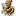•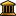•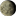•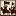•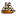•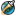•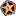•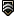•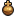•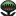•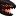••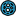•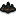•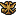•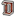•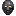•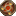•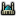•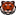•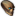•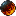•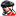••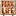•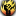•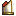•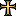•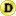•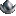•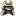•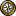•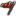•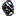•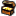•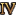•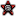•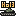•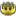•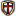••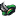•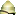•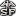•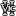•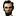•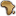•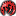•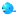•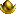Todor said:
There are two remaining issues which should be discussed: First, while German sources give the names of certain grandmasters with the land of their origion (e.g. "of Brandenburg-Ansbach", "of Österreich"), other languages tend to use dynasties instead (e.g. "of Hohenzollern", "of Habsburg"). I think we could go either way, but it should be uniform.

My personal preference would be to use dynasty instead of land of origin, it just sounds better and feels more historical (though seeing as german sources use land of origin, that is probably simply my personal feeling)

Todor said:
Second, the problem of Albrecht of Brandenburg-Ansbach/Hohenzollern. If a player decides not to secularize and stay as the order, Albrecht would not be deposed as grandmaster in 1525 and could - most likely - rule until his death in 1568. Therefore, two historical grandmasters between 1525 and 1568 have to be skipped from the list. I think this can not be avoided, as there was no reason for Albrecht to abdicate in 1525 if he chose not to convert to Protestantism.

On this I am somewhat divided: on the one hand your argument certainly makes sense; but on the other hand we really don't know who would have become the succesive grandmasters of a non-secularized and still landed teutonic order thus we just use the historic ones which should include the two eclipsed/overlapped by Albrecht.

on second thought, your proposal is probably the best compromise between just using the historical grandmasters and making up an entire new list of "more plausible ahistoric grandmasters"

#### unmerged(44390)

##### Second Lieutenant
I vote for dynasty.

#### Norrefeldt

##### Porphyrogenitus
I agree. It blends in better with those where there's no land to add, just a family name.

Me too.

#### unmerged(54293)

##### Second Lieutenant
Thanks for your help. The list is updated accordingly (note that "von Lothringen" and "von Pfalz-Neuburg" are also dynasties, I could not come up with something else).

#### unmerged(54293)

##### Second Lieutenant
Next, the Livonian Order. In the first step, a few spelling mistakes had to be corrected. Second, I decided to add some fantasy monarchs for the time after the Order's historical comedown in the 1560s, if someone (like me) fancies playing the Livonians until 1819. For this purpose, I collected some actual existing Baltic nobles and German members of the Teutonic Order from a genealogical handbook and Wikipedia - personalities of whom It's remotely possible they could have been members of the Order in an alternate history.

Things changed in the first part:
Above all, I had to alter the names of the Grandmasters and make their dates of reign more precise. My sources were worldstatesmen.org and the standard scholarly work on the topic (in German): Lutz Fenske: Ritterbrüder im livländischen Zweig des Deutschen Ordens [Knights in the Livonian branch of the Teutonic order]. Cologne 1993. Ordinal numbers were left out as they are absolutely uncommon for Grandmasters. Changes in stats were minor, save Wolter von Plettenberg, who had been completely underrated in vanilla version.

The changes in detail:
• 078000: "Siegried Lander von Spanheim" changed to "Siegfried Lander von Sponheim", specified his deathdate
• 078001: "Cysse von Rutenberg" changed to "Cisse von dem Rutenberg", specified his deathdate
• 078002: "Frank von Kersdorf" changed to "Frank Kirskorf", specified his deathdate
• 078003: "Heinrich III von Buckenorde" changed to "Heinrich von Böckenförde", specified his deathdate
• 078004: "Heinrich IV Vinke von Oberbergen" changed to "Heidenreich Vincke von Overberg", corrected his deathdate
• 078005: "Johann I von Mengden" changed to "Johann von Mengede", raised his DIP from 5 to 6 because of diplomatic success, specified his deathdate
• 078006: "Johann II Wolthusen von Heerse" changed to "Johann Waldhaus von Heerse", specified his deathdate
• 078007: "Bernhard von dem Borch" changed to "Bernhard von der Borch", specified his deathdate
• 078008: "Johann III Freitag von Loringhof" changed to "Johann Freitag von Loringhoven"
• 078009: "Walther II von Plettenberg" changed to "Wolter von Plettenberg", raised his abilities distinctly as he was one of the most successfull grandmasters
• 078010: "Hermann II Brugsenei" changed to "Hermann Brüggenei"
• 078011: "Johann IV von der Recke" changed to "Johann von der Recke"
• 078012: "Heinrich V von Galen" changed to "Heinrich von Galen", raised his ADM by 1 to reflect that he managed to hold the order together after the reformation
• 078013: "Wilhelm III von Fürstenberg" changed to "Johann Wilhelm von Fürstenberg", lowered his abilities a bit as his reign is considered a failure
• 078014: "Gotthard von Kettler" changed to "Gotthard Kettler", raised his DIP by 1 to reflect his skills in negotiating with the Polish king, extended his deathdate to his natural death (if the order is not secularized in 1562)

Here is my proposal:

Code:
``````# Livonian Order #

# Grandmasters of the Livonian Order. Original by Paradox, enhanced by Todor. #

historicalmonarch = {
id = { type = 6 id = 078000 }
name = "Siegfried Lander von Sponheim"
startdate = {
year = 1415
}
deathdate = {
day = 29  # 31th IRL
month = march
year = 1424
}
DIP = 4
MIL = 5
dormant = no
}
historicalmonarch = {
id = { type = 6 id = 078001 }
name = "Cisse von dem Rutenberg"
startdate = {
day = 29
month = march
year = 1424
}
deathdate = {
month = october
year = 1433
}
DIP = 3
MIL = 5
dormant = no
}
historicalmonarch = {
id = { type = 6 id = 078002 }
name = "Frank Kirskorf"
startdate = {
month = october
year = 1433
}
deathdate = {
day = 1
month = september
year = 1435
}
DIP = 4
MIL = 5
dormant = no
}
historicalmonarch = {
id = { type = 6 id = 078003 }
name = "Heinrich von Böckenförde"
startdate = {
day = 1
month = september
year = 1435
}
deathdate = {
month = december
year = 1437
}
DIP = 4
MIL = 5
dormant = no
}
historicalmonarch = {
id = { type = 6 id = 078004 }
name = "Heidenreich Vincke von Overberg"
startdate = {
month = december
year = 1437
}
deathdate = {
day = 29
month = june
year = 1450
}
DIP = 6
MIL = 5
dormant = no
}
historicalmonarch = {
id = { type = 6 id = 078005 }
name = "Johann von Mengede"
startdate = {
day = 29
month = june
year = 1450
}
deathdate = {
day = 15
month = august
year = 1469
}
DIP = 6
MIL = 5
dormant = no
}
historicalmonarch = {
id = { type = 6 id = 078006 }
name = "Johann Waldhaus von Heerse"
startdate = {
day = 15
month = august
year = 1469
}
deathdate = {
month = october
year = 1471
}
DIP = 5
MIL = 5
dormant = no
}
historicalmonarch = {
id = { type = 6 id = 078007 }
name = "Bernhard von der Borch"
startdate = {
month = october
year = 1471
}
deathdate = {
month = november
year = 1483
}
DIP = 5
MIL = 5
dormant = no
}
historicalmonarch = {
id = { type = 6 id = 078008 }
name = "Johann Freitag von Loringhoven"
startdate = {
month = november
year = 1483
}
deathdate = {
day = 26
month = may
year = 1494
}
DIP = 5
MIL = 4
dormant = no
}
historicalmonarch = {
id = { type = 6 id = 078009 }
name = "Wolter von Plettenberg"
startdate = {
day = 26
month = may
year = 1494
}
deathdate = {
day = 28
month = february
year = 1535
}
DIP = 8
MIL = 8
dormant = no
}
historicalmonarch = {
id = { type = 6 id = 078010 }
name = "Hermann Brüggenei"
startdate = {
day = 28
month = february
year = 1535
}
deathdate = {
day = 5
month = february
year = 1549
}
DIP = 4
MIL = 6
dormant = no
}
historicalmonarch = {
id = { type = 6 id = 078011 }
name = "Johann von der Recke"
startdate = {
day = 5
month = february
year = 1549
}
deathdate = {
day = 18
month = may
year = 1551
}
DIP = 4
MIL = 5
dormant = no
}
historicalmonarch = {
id = { type = 6 id = 078012 }
name = "Heinrich von Galen"
startdate = {
day = 18
month = may
year = 1551
}
deathdate = {
day = 29  # 30th IRL
month = may
year = 1557
}
DIP = 4
MIL = 5
dormant = no
}
historicalmonarch = {
id = { type = 6 id = 078013 }
name = "Johann Wilhelm von Fürstenberg"
startdate = {
day = 29
month = may
year = 1557
}
deathdate = {
day = 17
month = september
year = 1559
}
DIP = 3
MIL = 3
dormant = no
}
historicalmonarch = {
id = { type = 6 id = 078014 }
name = "Gotthard Kettler"
startdate = {
day = 17
month = september
year = 1559
}
deathdate = {
day = 17
month = may
year = 1587
}
DIP = 6
MIL = 5
dormant = no
remark = "Had the Order not lost its lands in 1562, Gotthard could have ruled as Grandmaster until his death in 1587."
}

# From 1587 on, fictional monarchs: Historical Baltic and German nobles, clergymen and members of the Teutonic Order. #

historicalmonarch = {
id = { type = 6 id = 078015 }
name = "Wilhelm von Efferen *"
startdate = {
day = 17
month = may
year = 1587
}
deathdate = {
year = 1613 }
DIP = 4
MIL = 6
dormant = no
remark = "Komtur of the Livonian Order, later a high ranking official in the duchy of Courland."
}
historicalmonarch = {
id = { type = 6 id = 078016 }
name = "Gottschalk Vincke *"
startdate = {
year = 1613
}
deathdate = {
year = 1616
}
DIP = 3
MIL = 4
dormant = no
remark = "Knight of the Livonian Order, afterwards a member of the nobility of Courland."
}
historicalmonarch = {
id = { type = 6 id = 078017 }
name = "Dietrich von Landsberg *"
startdate = {
year = 1616
}
deathdate = {
year = 1623
}
DIP = 6
MIL = 5
dormant = no
remark = "Komtur of the Teutonic Order in Cologne, a nephew of the Livonian knight Wilhelm von Landsberg."
}
historicalmonarch = {
id = { type = 6 id = 078018 }
name = "Mathias von der Recke *"
startdate = {
year = 1623
}
deathdate = {
year = 1638
}
DIP = 4
MIL = 7
dormant = no
remark = "General and judge in the duchy of Courland, a son of the Livonian Komtur Mathias von der Recke (I)."
}
historicalmonarch = {
id = { type = 6 id = 078019 }
name = "Magnus von Pahlen *"
startdate = {
year = 1638
}
deathdate = {
year = 1646
}
DIP = 4
MIL = 5
dormant = no
remark = "Livonian Landrat and officer in the Swedish Army."
}
historicalmonarch = {
id = { type = 6 id = 078020 }
name = "Otto von Mengden *"
startdate = {
year = 1646
}
deathdate = {
year = 1670
}
DIP = 7
MIL = 4
dormant = no
remark = "Constituted the institutions of Livonian self-governance."
}
historicalmonarch = {
id = { type = 6 id = 078021 }
name = "Christoph Bernhard von Galen *"
startdate = {
year = 1670
}
deathdate = {
day = 19
month = september
year = 1678
}
DIP = 8
MIL = 5
dormant = no
remark = "Prince-bishop of Münster, a son of the Erbmarschall of Courland, Dietrich von Galen."
}
historicalmonarch = {
id = { type = 6 id = 078022 }
name = "Gustav Clodt von Jürgensburg *"
startdate = {
day = 19
month = september
year = 1678
}
deathdate = {
year = 1682
}
DIP = 4
MIL = 5
dormant = no
remark = "Livonian Landrat and officer in the Swedish Army."
}
historicalmonarch = {
id = { type = 6 id = 078023 }
name = "Franz-Wilhelm von Fürstenberg *"
startdate = {
year = 1682
}
deathdate = {
day = 2
month = september
year = 1688
}
DIP = 4
MIL = 4
dormant = no
remark = "Teutonic komtur of Westphalia, built the commandry of Mühlheim."
}
historicalmonarch = {
id = { type = 6 id = 078024 }
name = "Thomas Nolde *"
startdate = {
day = 2
month = september
year = 1688
}
deathdate = {
day = 11
month = november
year = 1694
}
DIP = 4
MIL = 7
dormant = no
remark = "Officer in the army of Courland."
}
historicalmonarch = {
id = { type = 6 id = 078025 }
name = "Johann von Patkul *"
startdate = {
day = 11
month = november
year = 1694
}
deathdate = {
day = 11
month = october
year = 1707
}
DIP = 7
MIL = 5
dormant = no
remark = "Diplomat who promoted Livonian self-governance."
}
historicalmonarch = {
id = { type = 6 id = 078026 }
name = "Georg Levin von Nagel *"
startdate = {
day = 11
month = october
year = 1707
}
deathdate = {
year = 1712
}
DIP = 4
MIL = 4
dormant = no
remark = "Teutonic Komtur of Westphalia."
}
historicalmonarch = {
id = { type = 6 id = 078027 }
name = "Georg Gustav von Rosen *"
startdate = {
year = 1712
}
deathdate = {
year = 1714
}
DIP = 1
MIL = 5
dormant = no
remark = "Baltic noble, soldier and notorious dueler."
}
historicalmonarch = {
id = { type = 6 id = 078028 }
name = "Christian von Sachsen-Zeitz *"
startdate = {
year = 1714
}
deathdate = {
day = 23
month = august
year = 1725
}
DIP = 6
MIL = 4
dormant = no
remark = "Noble from Saxony, a member of the Teutonic Order and bishop in Hungary."
}
historicalmonarch = {
id = { type = 6 id = 078029 }
name = "Joachim von Vietinghoff *"
startdate = {
day = 23
month = august
year = 1725
}
deathdate = {
year = 1731
}
DIP = 4
MIL = 6
dormant = no
remark = "Landrat of Oesel."
}
historicalmonarch = {
id = { type = 6 id = 078030 }
name = "Damian Hugo von Schönborn *"
startdate = {
year = 1731
}
deathdate = {
day = 19
month = august
year = 1743
}
DIP = 5
MIL = 5
dormant = no
remark = "Prince-bishop of Speyer and member of the Teutonic Order."
}
historicalmonarch = {
id = { type = 6 id = 078031 }
name = "Heinrich zur Mühlen *"
startdate = {
day = 19
month = august
year = 1743
}
deathdate = {
day = 6
month = october
year = 1750
}
DIP = 6
MIL = 3
dormant = no
remark = "Baltic German noble and mayor of Reval from 1745."
}
historicalmonarch = {
id = { type = 6 id = 078032 }
name = "Johann von der Osten-Sacken *"
startdate = {
day = 6
month = october
year = 1750
}
deathdate = {
day = 17
month = august
year = 1755
}
DIP = 3
MIL = 5
dormant = no
remark = "Landrat and judge in Oesel."
}
historicalmonarch = {
id = { type = 6 id = 078033 }
name = "Caspar Anton von Belderbusch *"
startdate = {
day = 17
month = august
year = 1755
}
deathdate = {
day = 2
month = january
year = 1784
}
DIP = 8
MIL = 4
dormant = no
remark = "Confidant of the Grandmaster of the Teutonic Order and Prime Minister of Cologne."
}
historicalmonarch = {
id = { type = 6 id = 078034 }
name = "Bernhard Heinrich Stryk *"
startdate = {
day = 2
month = january
year = 1784
}
deathdate = {
day = 24
month = february
year = 1787
}
DIP = 3
MIL = 3
dormant = no
remark = "Judge in Livonia."
}
historicalmonarch = {
id = { type = 6 id = 078035 }
name = "Jacob Johann von Sievers *"
startdate = {
day = 24
month = february
year = 1787
}
deathdate = {
year = 1801
}
DIP = 8
MIL = 4
dormant = no
remark = "Russian diplomat and reformer of German Baltic descent."
}
historicalmonarch = {
id = { type = 6 id = 078036 }
name = "Peter von der Pahlen *"
startdate = {
year = 1801
}
deathdate = {
year = 1808
}
DIP = 6
MIL = 6
dormant = no
remark = "Russian diplomat and general of German Baltic descent."
}
historicalmonarch = {
id = { type = 6 id = 078037 }
name = "Ludwig von Vincke *"
startdate = {
year = 1808
}
deathdate = {
year = 1844
}
DIP = 5
MIL = 4
dormant = no
remark = "Prussian satesman and reformer."
}

#First free id: 078038``````

Last edited:

#### unmerged(40707)

##### Just call me Yoda in private!
could you please show us in yellow modifications you made before 1560?

It will help a lot.

#### unmerged(54293)

##### Second Lieutenant
Ah, I forgot. I added a list of the changes in detail instead.

For the names, they can be discussed of course, but I simply took what I found on "worldstatesmen" and the literature on this topic.

Thanks!

#### unmerged(54293)

##### Second Lieutenant
Pushing this one up a bit...

#### unmerged(40707)

##### Just call me Yoda in private!
This new list seems fine.

#### unmerged(40707)

##### Just call me Yoda in private!
Is there a reason why capital of Livonian Order is in Livland in revolt.txt:
Code:
``````LIV = { #Livonian Order
no = KUR
revolt = no
date = { day = 1 month = january year = 1419 }
expirydate = { day = 29 month = december year = 1561 }
minimum = { 282 }
extra = { 281 283 276 275 }
capital = 282
group = latin
culture = german
#ai = "Teutons.ai"
}``````
while capital of Livonian Order is in Kurland in 1419 and no change by events?

I found this very old post and here where setup in 1419 was for a capital in Livland (Riga).

Last edited:

#### Robmel

##### Second Lieutenant
•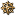••••••••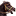•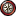••••••••••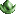•YodaMaster said:
Is there a reason why capital of Livonian Order is in Livland in revolt.txt:
Historically the capital of Livonian Order was Wenden (modern Cēsis) in the Livland province. The Livonian confederation landtag (parliament) came together in Walk (Valka) or Wolmar (Valmiera) in the same Livland province.

#### unmerged(40707)

##### Just call me Yoda in private!
Welcome and thanks for this long awaited answer. In this case, is there a reason for the capital to be in Kurland province in 1419? Or is it an error?

#### Robmel

##### Second Lieutenant
•••••••••••••••••••••YodaMaster said:
Welcome and thanks for this long awaited answer. In this case, is there a reason for the capital to be in Kurland province in 1419? Or is it an error?
There is no reason for the capital to be in Kurland province in 1419, because Livonian order didn't any large towns there. Mitau (the future capital of Duchy of Kurland, now modern Jelgava) at that time was just a mere village with an order's castle. It received town rights only in 1573.

Also Wenden town was the approximate geographical center of Livonian order possesions and the forth largest town behind Riga, Revel, Dorpat.

Thanks again.

#### Toio

##### Field Marshal
••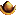•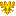•••What would be the culture of livonian order then , german which in our game in those parts is Baltic, so if you change capital in to another province, then baltic should replace ugric

or have capital baltic , province ugric

#### unmerged(40707)

##### Just call me Yoda in private!
Problem is just about capital location in 1419, not about culture and revolt.txt (see post #74, culture will be German if revolter and German + Baltic + Ugric in this order at start).
i'm not sure I understand the proposed change(s). Kurland province is Baltic and Livland province is Ugric. Where is the problem?